2019-12-06 21:29:25 qq_42369840 阅读数 96
• ###### 单片机控制第一个外设-LED灯-第1季第6部分

本课程是《朱有鹏老师单片机完全学习系列课程》第1季第6个课程，主要讲解LED的工作原理和开发板原理图、实践编程等，通过学习目的是让大家学会给单片机编程控制LED灯，并且为进一步学习其他外设打好基础。

4001 人正在学习 去看看 朱有鹏

## 一、实验目的

1、熟悉P1口作输出口的原理与方法
2、掌握循环延时，并能计算延时时间
3、能熟练的使用keil编程和proteus仿真
4、实现多个流水灯的控制

## 二、实验内容

1、用keil编写程序，编译运行
2、用proteus进行仿真检验
3、用面包板进行搭线展示，用2个流水灯实现流水效果

## 三、实验原理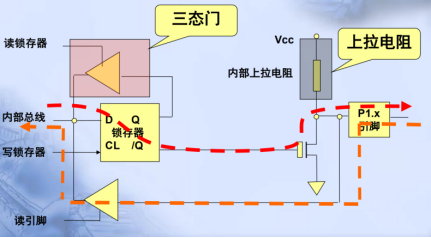## 四、电路图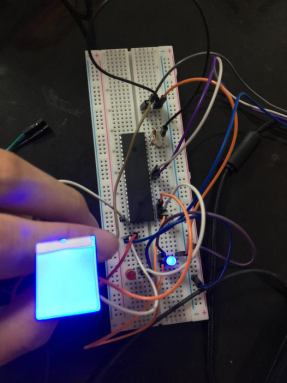proteus仿真结果：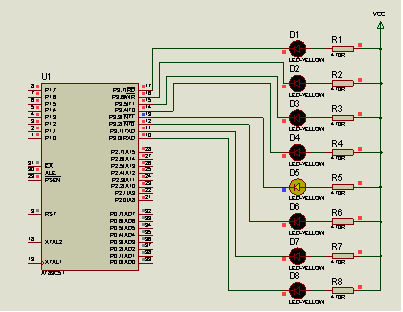## 五、流程图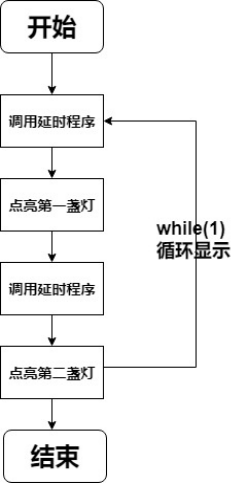## 六、源代码

``````#include <reg51.h>
sbit Led_0 = P1^0;
sbit Led_1 = P1^1;
sbit touch = P1^2;	//HTTM触摸开关

void delay()
{
int i,j;
for(i=0;i<200;i++)
for(j=0;j<200;j++);
}
void main()
{
touch = 0;
while(1)
{					   //说明：此按键为HTTM触摸开关
//按下为高电平，灯亮；不按下为低电平，灯灭
if(touch == 1)	  //有按键被按下
{
Led_0 = 1;
Led_1 = 0;
delay();
Led_0 = 0;
Led_1 = 1;
delay();
}
else 	//没有按键被按下
{
Led_0 = 1;
Led_1 = 1;
}
}
}
``````

## 七、流水灯进阶

1. 用C语言设计，如何计算延时时间
对于延时时间可以通过示波器进行准确的查看。在这里我准备了两段延时函数，分别是1ms，test(自定义)来进行测试，前面的1ms是经过精确计算无误的时间，后面的test则是自己需要计算的延时函数。
1ms测试结果（准确时间）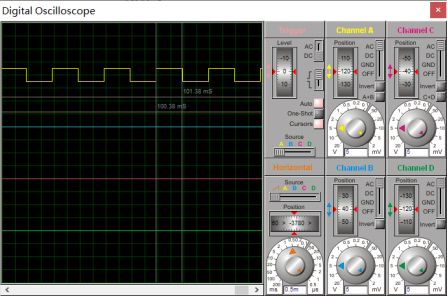测试结果：1ms时间准确。

Test延时函数测试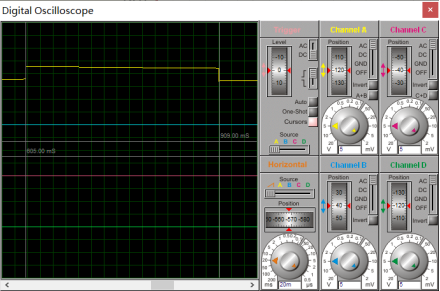Test延时函数测试结：delay = (909-605)ms = 304ms。

``````#include <reg51.h>
sbit Out = P1^0;

void Delay1ms(void)   //测试1ms 误差 0us
{
unsigned char a,b,c;
for(c=1;c>0;c--)
for(b=142;b>0;b--)
for(a=2;a>0;a--);
}
void Delay10ms(void)   //测试10ms 误差 0us
{
unsigned char a,b,c;
for(c=5;c>0;c--)
for(b=4;b>0;b--)
for(a=248;a>0;a--);
}
void Delay_Test(void) //测试结果304ms
{
int i,j;
for(i=0;i<250;i++)
for(j=0;j<150;j++);
}
void main()
{
while(1)
{
Out = 1;
Delay_Test();
Out = 0;
Delay_Test();
}
}
``````
1. 当LED的数量远多于接口数量时（如50，100，1000个），该怎么实现流水灯？
解答如下：实现方法较多，这里我采用的是3-8译码器，通过3-8译码器的级联可以实现led数目的增加，实现50，100个没有问题，如果要实现1000个则需要4-16译码器，并通过级联的方式实现。
实现结果如下：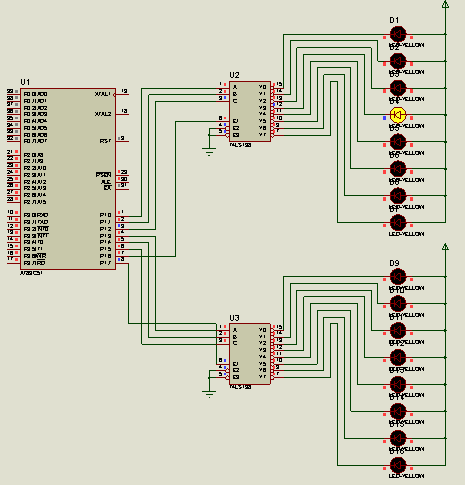源代码如下：
``````#include <reg51.h>
//用6个IO口点亮16个流水灯
//实现效果：可以用8个IO口点亮16个流水灯，
//分别为3 3显示 2片选，最多可控制16*4=64个
/*
如果采用4-16译码器，包括片选端，那么一个占8个位置，可以亮16*16=256
一共有32/8=4那么4*256=1024个灯，满足一千个灯
*/
sbit crol_1 = P1^0;
sbit crol_2 = P1^1;
sbit crol_3 = P1^2;	//设置控制代码
sbit crol_4 = P1^3;
sbit crol_5 = P1^4;
sbit crol_6 = P1^5;	//第二个译码器
sbit select_1 = P1^6;
sbit select_2 = P1^7;//片选
static int count = 0;

void delay_ms()	//设置延时函数
{
int i,j;
for(i=0;i<250;i++)
for(j=0;j<250;j++);
}
void Crol_Flow_1()  //前八个
{
select_1 = 1;	//设置片选端
select_2 = 0;
switch (count)
{
case 0:
crol_3 = 0,crol_2 = 0,crol_1 = 0;
break;
case 1:
crol_3 = 0,crol_2 = 0,crol_1 = 1;
break;
case 2:
crol_3 = 0,crol_2 = 1,crol_1 = 0;
break;
case 3:
crol_3 = 0,crol_2 = 1,crol_1 = 1;
break;
case 4:
crol_3 = 1,crol_2 = 0,crol_1 = 0;
break;
case 5:
crol_3 = 1,crol_2 = 0,crol_1 = 1;
break;
case 6:
crol_3 = 1,crol_2 = 1,crol_1 = 0;
break;
case 7:
crol_3 = 1,crol_2 = 1,crol_1 = 1;
break;
}
}
void Crol_Flow_2()  //后八个
{
select_1 = 0;	//片选端
select_2 = 1;
switch (count)  //设计亮的顺序
{
case 8:
crol_6 = 0,crol_5 = 0,crol_4 = 0;
break;
case 9:
crol_6 = 0,crol_5 = 0,crol_4 = 1;
break;
case 10:
crol_6 = 0,crol_5 = 1,crol_4 = 0;
break;
case 11:
crol_6 = 0,crol_5 = 1,crol_4 = 1;
break;
case 12:
crol_6 = 1,crol_5 = 0,crol_4 = 0;
break;
case 13:
crol_6 = 1,crol_5 = 0,crol_4 = 1;
break;
case 14:
crol_6 = 1,crol_5 = 1,crol_4 = 0;
break;
case 15:
crol_6 = 1,crol_5 = 1,crol_4 = 1;
break;
}
}
void main()
{
while(1)
{
if(count == 16)
count = 0; //若是等于16则清零
else if(count < 8)
{
Crol_Flow_1();
delay_ms();
count++;
}
else if (count < 16)
{
Crol_Flow_2();
delay_ms();
count++;
}
}
}
``````

## 八、说点什么

2019.12.6

2019-08-24 14:38:33 qq_45400902 阅读数 488
• ###### 单片机控制第一个外设-LED灯-第1季第6部分

本课程是《朱有鹏老师单片机完全学习系列课程》第1季第6个课程，主要讲解LED的工作原理和开发板原理图、实践编程等，通过学习目的是让大家学会给单片机编程控制LED灯，并且为进一步学习其他外设打好基础。

4001 人正在学习 去看看 朱有鹏

``````#include<reg52.h>
#include<intrins.h>            //包含_crol_和__cror_函数所在头文件
#define uint unsigned int
#define uchar unsigned char
sbit beep=P2^3;               //设置蜂鸣器所连接端口
void delay(uint y);           //声明延迟函数
void main()
{
while(1)
{
int x;
P1=0xfe;           //初始状态11111110，右数第一个灯低电平，状态为点亮
for(x=7;x>0;x--)
{
beep=0;
delay(300);
P1=_crol_(P1,1);      //每循环一次，0的位置左移一位
beep=1;
delay(300);
}
P1=0x7f;                  //左数第一个灯点亮状态，点亮的灯依次右移
for(x=7;x>0;x--)
{
beep=0;
delay(300);
P1=_cror_(P1,1);
beep=1;
delay(300);
}
P1=0x00;                  //所有的灯全部点亮
for(x=4;x>0;x--)          //全部熄灭，再全部点亮，重复四次
{
delay(200);
P1=0xff;
delay(200);
P1=0x00;
}
}
}

void delay(uint y)             //延迟函数
{
uint i,j;
for(i=100;i>0;i--)
for(j=y;j>0;j--);
}
``````

• ###### 单片机控制第一个外设-LED灯-第1季第6部分

本课程是《朱有鹏老师单片机完全学习系列课程》第1季第6个课程，主要讲解LED的工作原理和开发板原理图、实践编程等，通过学习目的是让大家学会给单片机编程控制LED灯，并且为进一步学习其他外设打好基础。

4001 人正在学习 去看看 朱有鹏

### 单片机 流水灯

通过Proteus仿真单片机最小系统+流水灯的显示电路。用Keil编写相应执行文件。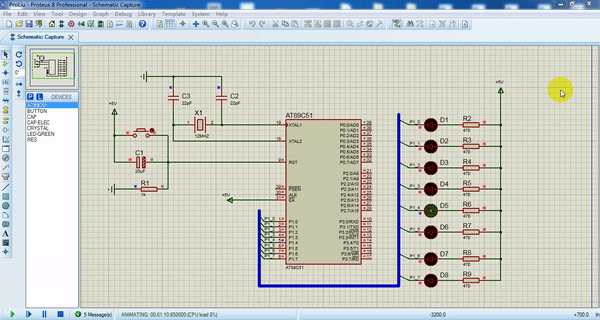1、Keil编程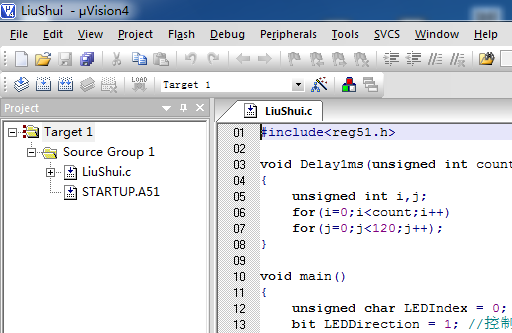2、仿真电路图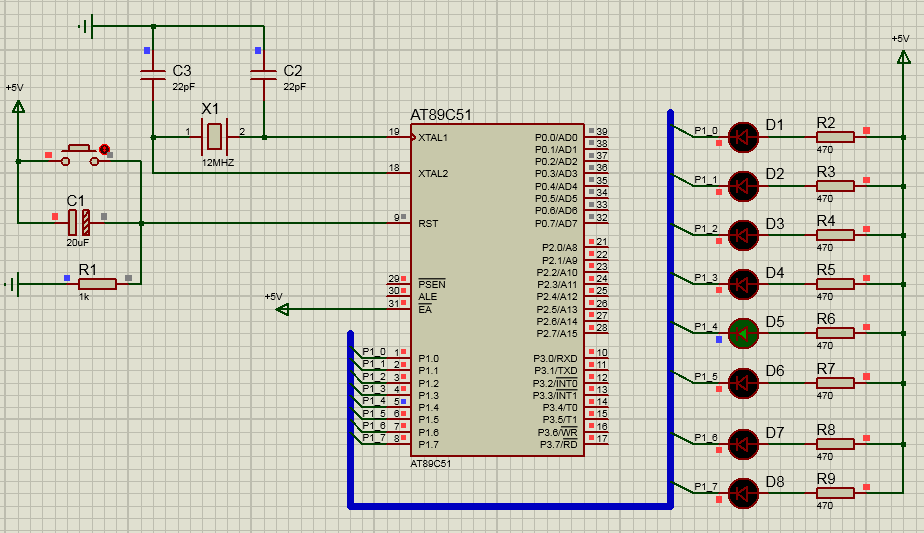LiuShui.c文件

``````#include<reg51.h>

//void Delay1ms(unsigned int count) //软延时count毫秒
//{
//	unsigned int i,j;
//	for(i=0;i<count;i++)
//	for(j=0;j<120;j++);
//}

void Delay1ms(unsigned int count)//延时count*50ms
{
unsigned int i;
TMOD=0x01;			   //选用定时器0且工作在方式1
TH0=0x3c;			  //设置初值0x3cb0
TL0=0xb0;
TR0=1;      //开启定时器
for(i=0;i<count;i++) //循环count次，每次定时50ms,总共count*50ms
{
while(TF0!=1);// 每次定时50ms (溢出时才会跳出循环)
TH0=0x3c;	 //重置初值0x3cb0
TL0=0xb0;
TF0=0;		   //软置溢出位
}
TR0=0;      //关闭定时器
}

void main()
{
unsigned char LEDIndex = 0;	  //指示第几个灯
bit LEDDirection = 1; //控制流水灯依次亮灭的方向

while(1)
{
if(LEDDirection)			//正方向，灯由上往下亮灭
P1 = ~(0x01<<LEDIndex);	  //P1寄存器控制P1并口	 电压为0时灯亮，先将要亮灯的某一位置1，其余置0，然后取反，就变为只有指定位为0，然后移位实现其他位置0
else
P1 = ~(0x80>>LEDIndex);
if(LEDIndex==7)
LEDDirection = !LEDDirection;	//到了最后一盏灯，改变灯的亮灭方向
LEDIndex = (LEDIndex+1)%8;	 //	  每次加一，%8保证 LEDIndex	取值在0~7之间
Delay1ms(2); //延时100ms
}
}
``````

选用单片机的P1端口8只引脚作为输出脚，1只引脚控制1只LED灯。设置P1_0脚为低电平，使得第1只LED灯点亮，并延时一点时间，然后设置P1_0脚为高电平，熄灭第1只LED，以此同理控制其余7只引脚。比如：

11111110   P1_0脚为低电平，使得第1只LED灯点亮

11111101   P1_1脚为低电平，使得第2只LED灯点亮

11111011   P1_2脚为低电平，使得第3只LED灯点亮

11110111   P1_3脚为低电平，使得第4只LED灯点亮

11101111   P1_4脚为低电平，使得第5只LED灯点亮

11011111   P1_5脚为低电平，使得第6只LED灯点亮

10111111   P1_6脚为低电平，使得第7只LED灯点亮

01111111   P1_7脚为低电平，使得第8只LED灯点亮

P1寄存器有8位，分别控制对应的8个引脚。

1、编写执行程序

(1)新建Project  (默认操作即可)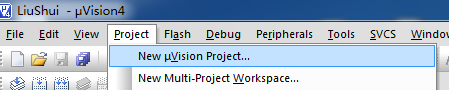(2)选择cpu型号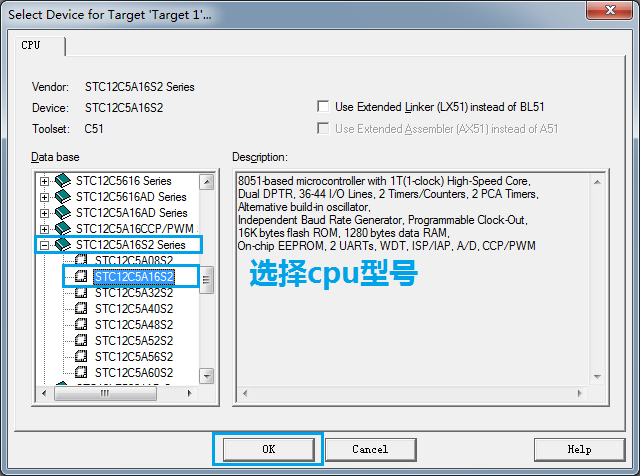(3)设置Project  属性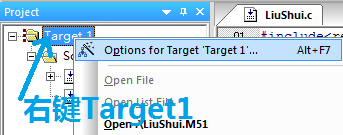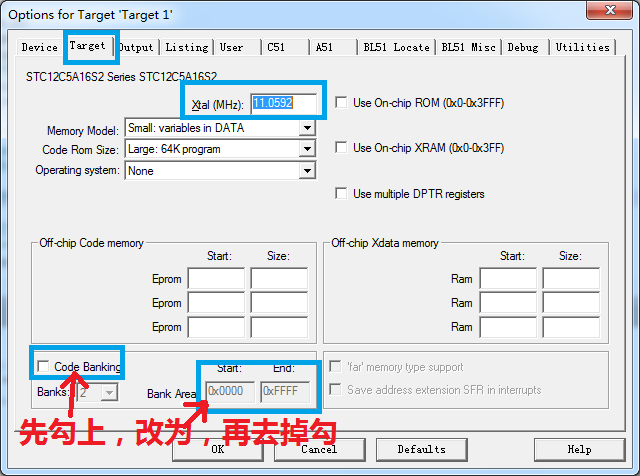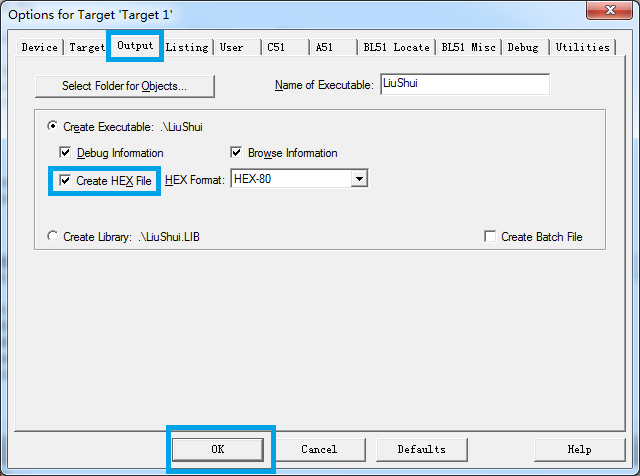(4)新建C文件，编写相应代码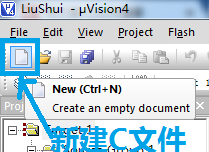(5)将C文件添加到工程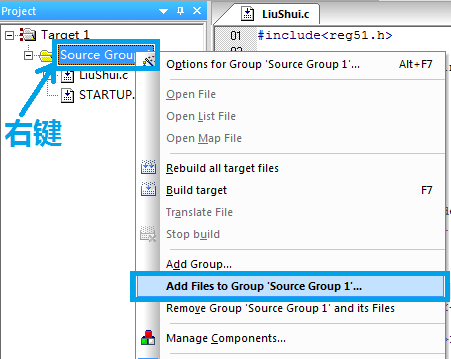(6)编译生成hex文件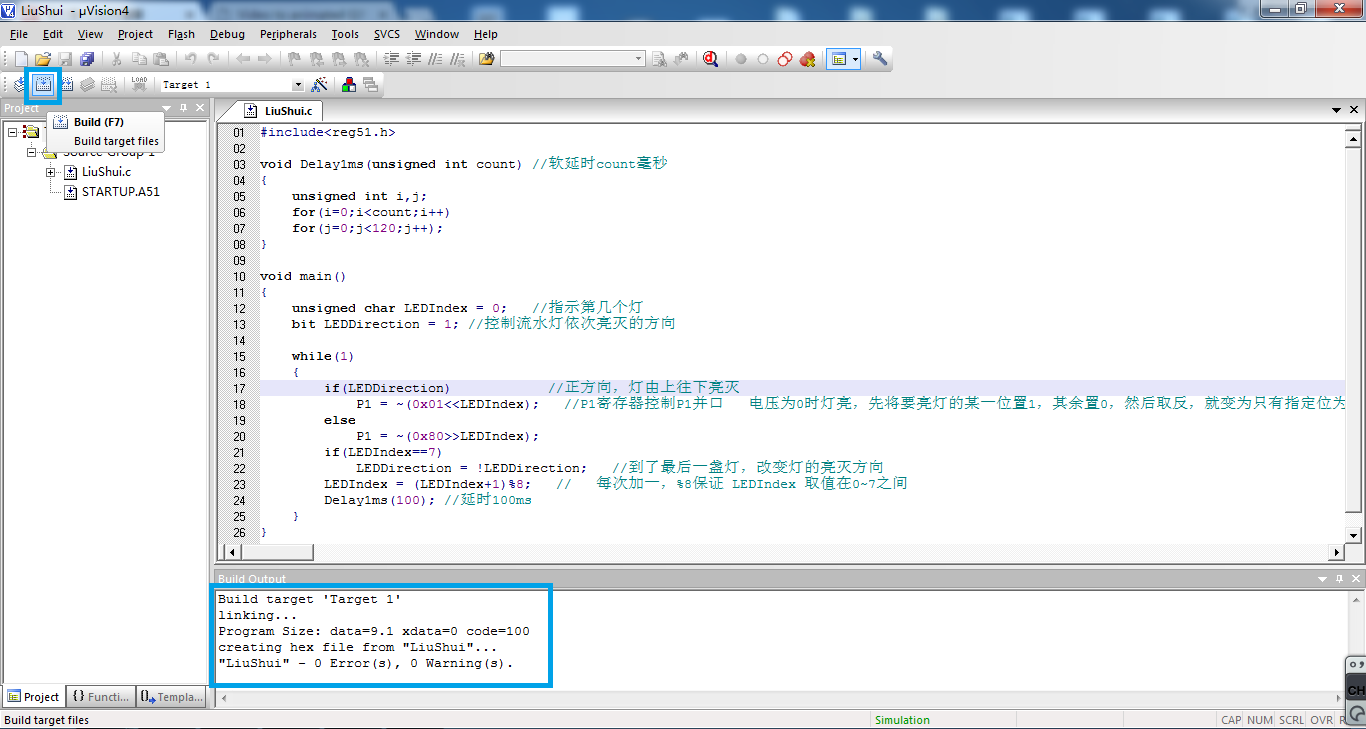2、仿真电路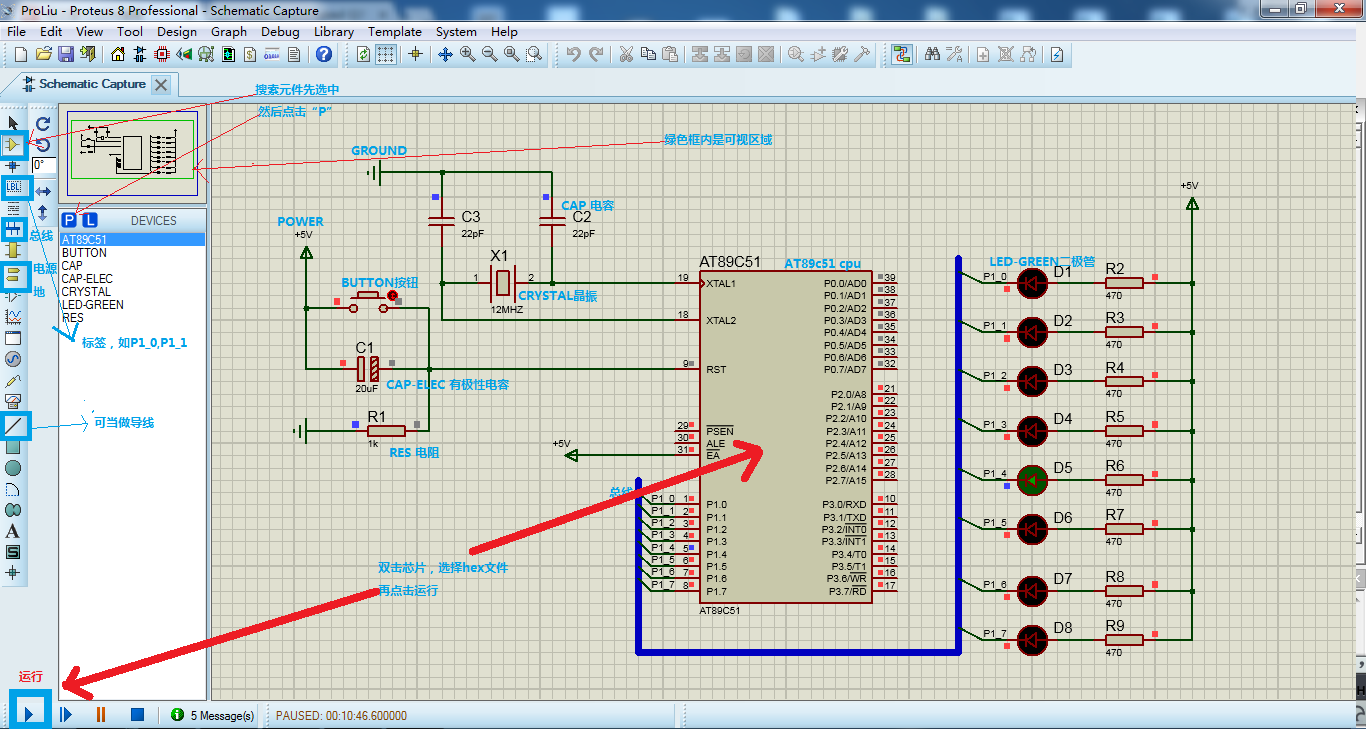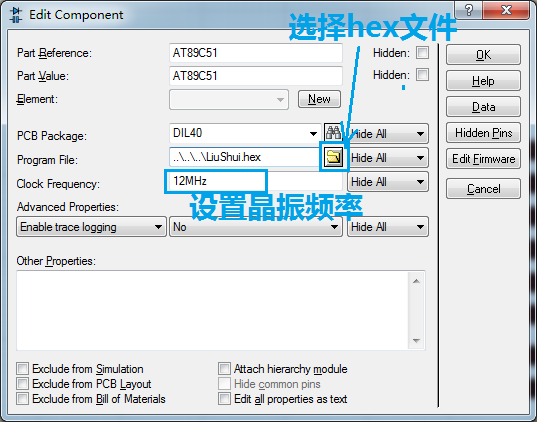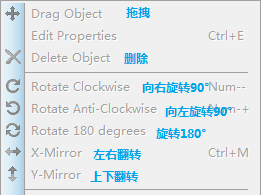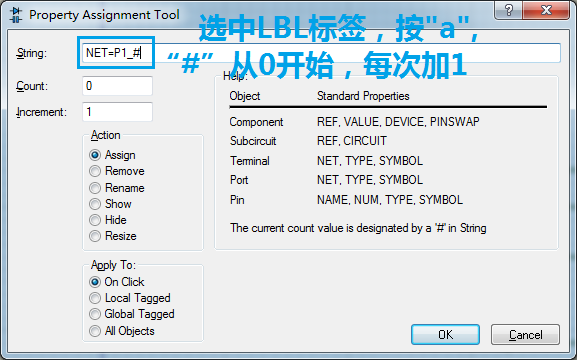=================================回复 weixin_44582500 这位兄弟=============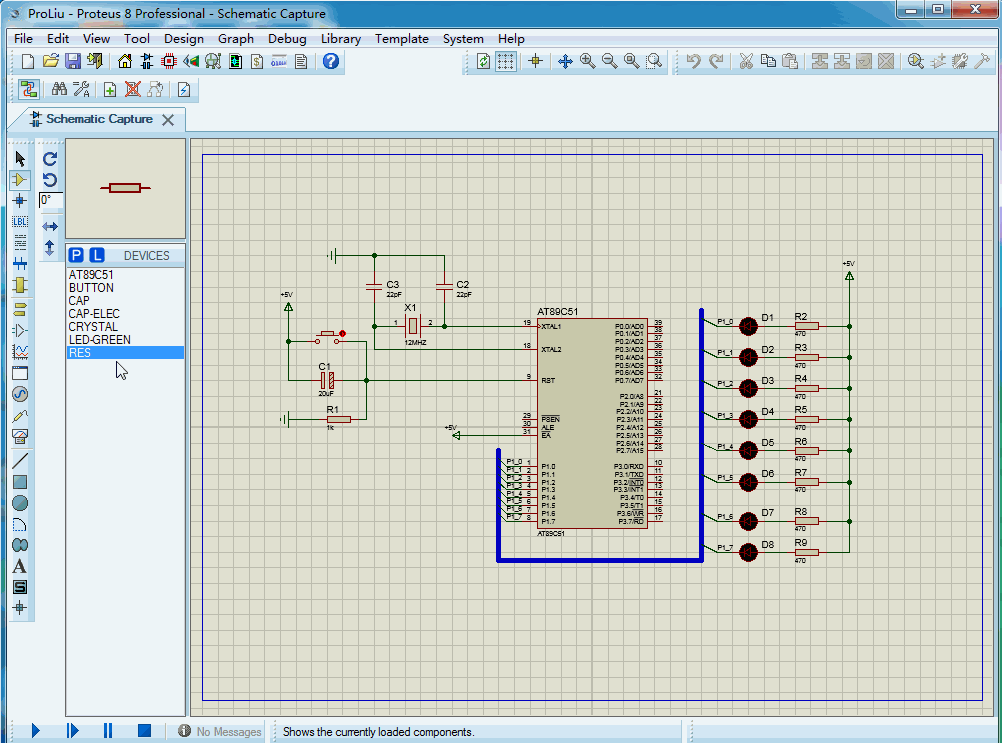2015-12-19 18:10:31 Tifa_Best 阅读数 3009
• ###### 单片机控制第一个外设-LED灯-第1季第6部分

本课程是《朱有鹏老师单片机完全学习系列课程》第1季第6个课程，主要讲解LED的工作原理和开发板原理图、实践编程等，通过学习目的是让大家学会给单片机编程控制LED灯，并且为进一步学习其他外设打好基础。

4001 人正在学习 去看看 朱有鹏

# 51单片机流水灯制作

## 初试与复试

### 复试

• 设计并制作基于 51 单片机的流水灯，通过按键实现 LED 灯点亮模
式的切换。
• 用 DXP 画出附录原理图， 并保存为.SchDoc 格式。

• 基本要求：（ 80 分）
• 所搭建的板子至少 8 个 LED 灯， 4 个按键，可实现至少四种 LED
点亮模式的变换。（ 50 分）
• 上交一份设计报告， 报告内容包括组元分工、硬件及软件设计，另需在附录附上完整程序。（ 20 分）
• 上交附录原理图。（ 10 分）
• 发挥部分：（ 20 分）
• 电路布局美观， 无虚焊， 焊点光度锥度好。
• 程序运行稳定。
• 其他。

## 制作

### 制作之软件

• 用一个定时器定时，如以100μs为一个小周期，然后以100个小周期为一个PWM周期（10ms），频率为100Hz（1s / 10 ms）。在定时器中断处理函数里直接输出buffer中的数据，或者在处理函数中处理判断后输出数据。
• 用一个定时器定时，比如给通电时间（占空比 * PWM周期）定时，然后在中断处理函数中启动不通电时间（PWM周期 - 通电时间）的定时，可用原来的定时器也可用其他的定时器。
• 用一个定时器给PWM周期定时，然后用另外一个定时器给通电时间（也可以是不通电时间）定时。
综合考虑后我选择第一种方法，噩梦才刚刚开始。
如何实现不同相位的多个平行正弦波的呼吸灯？使用小周期只是提供了实现的可能而已，还不够。后来在操场上散步时，才想明白了实现方式。我靠自己想明白了单极性脉宽调制的原理，相当激动啊。换句话说，我是使用单极性脉宽调制来实现想法的。设置一个数组来存储八个LED的占空比，占空比在时间维度上不断的沿正弦函数变化，在每个小周期的定时器中断时通过占空比与小周期计数的大小比较来确定引脚输出
然而实际效果不尽人意，几乎就是随意的亮灭。后来我通过keil的仿真是才发现，51单片机的计算能力太差，中断处理函数结束时，下一次中断几乎就出来了。对八个占空比的处理不能在完整的一次完成。几天的努力还是没能实现的自己当初的想法，有些失落，虽然自己收获了不少，特别是感觉自己对c语言小技巧的使用（有些自大啦啦啦）。
后来也就没办法，只好不用正弦波，只用三角波了。三角波也能玩出新花样。比如两排强度互补的呼吸灯。其实就是在传统意义上的不通电时间时输出对在通电时期输出数据的取反，比如在通电时间是输出0x00，在不通电时间输出0xFF。

## 总结

``````#include <STC89C5xRC.H>
#include<intrins.h>
#include<math.h>
#include<stdlib.h>

/*************全局常量***********/
#define uchar unsigned char
#define uint unsigned int
#define LED P1

/*****************全局变量**********************************/
uchar changed, status_switch = 0x00, status_switch_pre = 0x00;	//status_switch记录当前开关状态，status_switch_pre记录此前开关状态，changed记录开关状态是否变化
uchar counter_PWM = 0, length_PWM = 100;	//定时器0的两次中断时间为一小周期，length_PWM个小周期组成一个PWM大周期，counter_PWM负责计数，表示进行到了PWM大周期中的第counter_PWM个小周期
uchar duty_cycle, buffer;	//duty_cycle是占空比，buffer缓冲LED点亮信息

uchar i, j;
float f;

/*******************部分流水灯模式所需信息**************/
uchar code table1[]={0xfe,0xfc,0xf8,0xf0,0xe0,0xc0,0x80,0x00,0x80,0xc0,0xe0,0xf0,0xf8,0xfc,0xfc,0xff};
//0xfe,0xfd,0xfb,0xf7,0xef,0xdf,0xbf,0x7f	左移
uchar code table2[]={0x7f,0xbf,0xdf,0xef,0xf7,0xfb,0xfd,0xfe,0xfd,0xfb,0xf7,0xef,0xdf,0xbf};
uchar code table3[]={0x7e,0xbd,0xdb,0xe7,0xff};
uchar code table4[]={0xaa,0x55};

/*********函数声明********************/
void delay1ms();
void delay(uint x);
void keyscan();
void close_int();
void init_pwm();
void timer0_init(void);

/********主函数****************************/
void main()
{
while(1) {
keyscan();

switch (status_switch) {	//根据status_switch选择灯的点亮模式
case 0x00:
LED = 0xFF;
break;
case 0x01:
for (i=0;i<16;i++) {
LED=table1[i];
delay(200);
keyscan();
if (changed)
break;
}
break;
case 0x02:
for(i=0;i<14;i++) {
LED=table2[i];
delay(300);
keyscan();
if (changed)
break;
}
break;
case 0x03:
for(i=0;i<5;i++) {
LED=table3[i];
delay(250);
keyscan();
if (changed)
break;
}
break;
case 0x04:
for(i=0;i<2;i++){
LED=table4[i];
delay(3000);
keyscan();
if (changed)
break;
}
break;
case 0x05:
for (i=0;i<16;i++) {
LED=~table1[i];
delay(200);
keyscan();
if (changed)
break;
}
break;

case 0x06:
for(i=0;i<14;i++) {
LED=~table2[i];
delay(500);
keyscan();
if (changed)
break;
}
break;
case 0x07:
for(i=0;i<5;i++) {
LED=~table3[i];
delay(250);
keyscan();
if (changed)
break;
}
break;

case 0x08:
case 0x09:
case 0x0A:
case 0x0B:
timer0_init();
while (1) {
init_pwm();
for (i = 100; i > 0; i-=5) {
duty_cycle = i;				//改变占空比
delay(25);
keyscan();					//开关检测
if (changed) {
close_int();			//关闭定时器
break;					//退出for语句
}
}
break;							//退出switch语句
for (i = 0; i < 100; i+=5) {
duty_cycle = i;
delay(25);
keyscan();					//在该case语句中设置两个keyscan是为了更快的对开关变化做出响应
if (changed) {
close_int();
break;
}
}
break;
}
break;

case 0x0C:
case 0x0D:
timer0_init();
while (1) {
init_pwm();
delay(250);			//因为不需要改变有时间维度上的变化，所以没有占空比的改变，只需延时即可

keyscan();
if (changed) {
close_int();
break;
}
}
break;

case 0x0E:
srand(counter_PWM);	 //设置随机数种子
while(1) {
LED = rand() % 256;		//保证随机数在0与255之间
delay(200);

keyscan();
if (changed)
break;
}

break;
case 0x0F:
LED = 0x00;
break;
}
}
}

/*****************延时1ms*************/
void delay1ms()		//@11.0592MHz
{
unsigned char i, j;

_nop_();
i = 2;
j = 199;
do
{
while (--j);
} while (--i);
}

/**********延时x毫秒***********/
void delay(uint x)
{   uchar k;
for(k=x; k>0; k--)
delay1ms();
}

/****开关检测。修改开关状态(status_switch)以及更改标志（changed）****/
void keyscan()
{
status_switch_pre = status_switch;			//记录此前的开关状态
P2 = 0xFF;									//准备读取P2
status_switch = (~P2) & 0x0F;				//读取P2并提取有用信息
changed = (status_switch != status_switch_pre);			//判断是否改变
}

/*****关闭定时器0***********************/
void close_int()
{
TR0 = 0;//关闭Timer0
ET0 = 0;//关闭T0中断
LED = 0xFF;//PWM输出高电平
}

/*************PWM的初始化******/
void init_pwm()
{
buffer = 0x00;		//清空缓存区

duty_cycle = 0;		//归零占空比
}

/*****************定时器0************************/
void timer0_init(void)		//100微秒@11.0592MHz
{
AUXR &= 0x7F;		//定时器时钟12T模式
TMOD &= 0xF0;		//设置定时器模式
TMOD |= 0x02;		//设置定时器模式
TL0 = 0xA4;		//设置定时初值
TH0 = 0xA4;		//设置定时重载值
TF0 = 0;		//清除TF0标志
TR0 = 1;		//定时器0开始计时

ET0=1;			//开启T0中断
EA=1;			//开总中断
}

/*************定时中断1处理程序****************/
void timer0_int(void) interrupt 1
{

switch (status_switch) {
case 0x08:
/**********单个灯光强度在时间维度上呈三角形波*********/
buffer = 0x00;
buffer |= (counter_PWM < duty_cycle) << 0;
break;
case 0x09:
/*****两半灯光强度在空间维度上呈互补三角形波**************************/
if (counter_PWM < duty_cycle)
buffer = 0xF0;
else
buffer = 0x0F;
break;
case 0x0A:
/*****交叉灯的灯光强度在空间维度上呈互补三角形波**************************/
if (counter_PWM < duty_cycle)
buffer = 0x55;
else
buffer = 0xAA;
break;
case 0x0B:
/*****全部灯光强度在时间维度上呈三角形波***************/
if (counter_PWM < duty_cycle)
buffer = 0xFF;
else
buffer = 0x00;
break;

case 0x0C:
/***************灯光强度在空间维度上呈中凸三角形波************/
if (counter_PWM < 5)				//原谅我不是完美的三角形波
buffer = 0xFF;
else if (counter_PWM < 20)
buffer = 0x7E;
else if (counter_PWM <45)
buffer = 0x3C;
else
buffer = 0x18;
break;
case 0x0D:
/********灯光强度在空间维度上呈中凹三角形波******************/
if (counter_PWM < 5)
buffer = _crol_(0xFF, 4);
else if (counter_PWM < 20)
buffer = _crol_(0x7E, 4);
else if (counter_PWM <45)
buffer = _crol_(0x3C, 4);
else
buffer = _crol_(0x18, 4);
break;
}

LED = ~buffer;					//引脚的1代表灯灭，所以反转一下

if (counter_PWM >= length_PWM)
counter_PWM = 0;			//将counter_PWM限制在0到length_PWM之间
counter_PWM++;
}
``````

``````#include <STC89C5xRC.H>
#include<intrins.h>
#include<math.h>

/*************全局常量***********/
#define uchar unsigned char
#define uint unsigned int
#define LED P1
#define PI 3.1415926

uchar i, j;
float f;

/*****************全局变量**********************************/
uchar changed, status_switch = 0x00, status_switch_pre = 0x00;
uchar counter_PWM = 0, length_PWM = 100, buffer;
uchar duty_cycles;

/*******************部分流水灯模式所需信息**************/
uchar code table1[]={0xfe,0xfc,0xf8,0xf0,0xe0,0xc0,0x80,0x00,0x80,0xc0,0xe0,0xf0,0xf8,0xfc,0xfc,0xff};
//0xfe,0xfd,0xfb,0xf7,0xef,0xdf,0xbf,0x7f	左移
uchar code table2[]={0x7f,0xbf,0xdf,0xef,0xf7,0xfb,0xfd,0xfe,0xfd,0xfb,0xf7,0xef,0xdf,0xbf};
uchar code table3[]={0x7e,0xbd,0xdb,0xe7,0xff};
uchar code table4[]={0xaa,0x55};

/*********函数声明********************/
void delay1ms();
void delay(uint x);
void keyscan();
void close_int();
void init_pwm();
void timer0_init(void);

/********主函数*************/
void main()
{
while(1) {
keyscan();

switch (status_switch) {
case 0x00:
LED = 0xFF;
break;
case 0x01:
for (i=0;i<16;i++) {
LED=table1[i];
delay(200);
keyscan();
if (changed)
break;
}
break;
case 0x02:
for(i=0;i<14;i++) {
LED=table2[i];
delay(300);
keyscan();
if (changed)
break;
}
break;
case 0x03:
for(i=0;i<5;i++) {
LED=table3[i];
delay(250);
keyscan();
if (changed)
break;
}
break;
case 0x04:
for(i=0;i<2;i++){
LED=table4[i];
delay(300);
keyscan();
if (changed)
break;
}
break;
case 0x05:
for (i=0;i<16;i++) {
LED=~table1[i];
delay(200);
keyscan();
if (changed)
break;
}
break;

case 0x06:
for(i=0;i<14;i++) {
LED=~table2[i];
delay(300);
keyscan();
if (changed)
break;
}
break;

case 0x07:
for(i=0;i<5;i++) {
LED=~table3[i];
delay(250);
keyscan();
if (changed)
break;
}
break;

case 0x08:
/**********灯光强度在时间维度上呈三角形波*********/
timer0_init();
while (1) {
init_pwm();

for (i = 100; i > 0; i--) {
duty_cycles[j] = i;
buffer |= ((counter_PWM < duty_cycles[j]) << j);   //j代表第几盏灯，第一盏灯时j=0,因为没有指明j，所以每次都有不同的灯作为呼吸灯亮
delay(50);
keyscan();
if (changed) {			 //在此插入changed的判断语句是为了更好的对开关变化做出响应
close_int();
break;
}
}
for (i = 0; i < 100; i++) {
duty_cycles[j] = i;
buffer |= ((counter_PWM < duty_cycles[j]) << j);   //j代表第几盏灯，第一盏灯时j=0;
delay(50);
keyscan();
if (changed) {
close_int();
break;
}
}
}
break;
case 0x09:
/***************灯光强度在空间维度上呈三角形波************/
timer0_init();
for (j = 0; j < 4; j++)
duty_cycles[j] = 25 * j;
for (j = 4; j < 8; j++)
duty_cycles[j] = 25 * (8 - j);

while (1) {
init_pwm();
for (j = 0; j < 8; j++) {
buffer |= ((counter_PWM < duty_cycles[j]) << j);
}
// 					if (counter_PWM < 25)
//						buffer = 0xFF;
//					else if (counter_PWM < 50)
//						buffer = 0x7E;
//					else if (counter_PWM < 75)
//						buffer = 0x3C;
//					else
//						buffer = 0x18;
delay(100);

keyscan();
if (changed) {
close_int();
break;
}
}
break;
case 0x0A:
/********灯光强度在时间、空间维度上呈三角形波******************/
timer0_init();
while (1) {
init_pwm();
for (i = 0; i < 100; i++) {
for (j = 0; j < 4; j++)
duty_cycles[j] = (25 * j + i) % 100;
for (j = 4; j < 8; j++)
duty_cycles[j] = (25 * (8 - j) + i) % 100;
for (j = 0; j < 8; j++)
buffer |= ((counter_PWM < duty_cycles[j]) << j);
delay(50);

keyscan();
if (changed) {
close_int();
break;
}
}
for (i = 100; i > 0; i--) {
for (j = 0; j < 4; j++)
duty_cycles[j] = (25 * j + i) % 100;
for (j = 4; j < 8; j++)
duty_cycles[j] = (25 * (8 - j) + i) % 100;
for (j = 0; j < 8; j++)
buffer |= ((counter_PWM < duty_cycles[j]) << j);
delay(50);

keyscan();
if (changed) {
close_int();
break;
}
}
}
break;
case 0x0B:
/*****灯光强度在时间维度上呈正弦***************/
timer0_init();
while (1) {
init_pwm();

for (i = 0; i < 180; i++) {
duty_cycles[j] = (uchar)(sin(PI * i / 180) * 100);	//j号灯为呼吸灯
buffer |= ((counter_PWM < duty_cycles[j]) << j);
delay(50);

keyscan();
if (changed) {
close_int();
break;
}
}
}
break;
case 0x0C:
/*****灯光强度在空间维度上呈正弦波**************************/
timer0_init();
while (1) {
init_pwm();
for (j = 0; j < 8; j++) {
duty_cycles[j] = (uchar)(sin(PI * ((int)(i + 22.5 * j) % 180) /180) * 100);
buffer |= (counter_PWM < duty_cycles[j]) << j;
}
delay(100);

keyscan();
if (changed) {
close_int();
break;
}
}
break;
case 0x0D:
/**灯光强度在时间维度上呈三角形波，空间上为相位相差22.5度的数个平行正弦波**/
timer0_init();
while (1) {
init_pwm();
for (i = 0; i < 180; i++) {
buffer = 0x00;
for (j = 0; j < 8; j++) {
duty_cycles[j] = (uchar)(sin(PI * ((int)(i + 22.5 * j) % 180) /180) * 100);
buffer |= (counter_PWM < duty_cycles[j]) << j;
}
delay(10);

keyscan();
if (changed) {
close_int();
break;
}
}
}
break;
case 0x0E:
/*灯光强度在时间维度上呈正弦波，空间上为强度相差22级的平行正弦波*/
timer0_init();
while (1) {
for (i = 0; i < 180; i++) {
buffer = 0x00;
for (j = 0; j < 8; j++) {
//						duty_cycles[j] = (uchar)((int)(sin(PI * i / 180) * 100 + 22) % 100);
duty_cycles[j] = (uchar)(sin(PI * i / 180) * 100 + 22);

buffer |= (counter_PWM < duty_cycles[j]) << j;
}
delay(10);

keyscan();
if (changed) {
close_int();
break;
}
}
}
break;
case 0x0F:
LED = 0x00;
break;
}
}
}

/*****************延时1ms*************/
void delay1ms()		//@11.0592MHz
{
unsigned char i, j;

_nop_();
i = 2;
j = 199;
do
{
while (--j);
} while (--i);
}

/**********延时x毫秒***********/
void delay(uint x)
{   uchar k;
for(k=x; k>0; k--)
delay1ms();
}

/****开关检测。修改开关状态(status_switch)以及更改标志（changed）****/
void keyscan()
{
status_switch_pre = status_switch;
P2 = 0xFF;
status_switch = (~P2) & 0x0F;
changed = (status_switch != status_switch_pre);
}

/*****关闭定时器0***********************/
void close_int()
{
TR0 = 0;//关闭Timer0
ET0 = 0;//关闭T0中断
LED = 0xFF;//PWM输出高电平
}

/*************PWM的初始化******/
void init_pwm()
{
uchar k;

buffer = 0x00;
for (k = 0; k < 8; k++)
duty_cycles[k] = 0;
}

/*****************定时器0************************/
void timer0_init(void)		//100微秒@11.0592MHz
{
AUXR &= 0x7F;		//定时器时钟12T模式
TMOD &= 0xF0;		//设置定时器模式
TMOD |= 0x02;		//设置定时器模式
TL0 = 0xA4;		//设置定时初值
TH0 = 0xA4;		//设置定时重载值
TF0 = 0;		//清除TF0标志
TR0 = 1;		//定时器0开始计时

ET0=1;			//开启T0中断
EA=1;			//开总中断
}

/*************定时中断1处理程序****************/
void timer0_int(void) interrupt 1
{
LED = ~buffer;					//引脚的1代表灯灭，所以反转一下

if (counter_PWM >= length_PWM)
counter_PWM = 0;			//将counter_PWM限制在0到length_PWM之间
counter_PWM++;
}
``````

2015-08-02 18:20:27 yunfeiyang62 阅读数 2392
• ###### 单片机控制第一个外设-LED灯-第1季第6部分

本课程是《朱有鹏老师单片机完全学习系列课程》第1季第6个课程，主要讲解LED的工作原理和开发板原理图、实践编程等，通过学习目的是让大家学会给单片机编程控制LED灯，并且为进一步学习其他外设打好基础。

4001 人正在学习 去看看 朱有鹏
51单片机 流水灯 蜂鸣器应用
1. 闹铃小程序：
```/*======================================================*/
/*	时间：2015年8月2日 16:42:05							*/
/*	功能：蜂鸣器1：闹钟 								*/
/*	目的：51单片机学习 									*/
/*		^_^……！											*/
/*======================================================*/

#include
#include "commLib.h"

int main(void)
{
unsigned int cnt;

while(1)
{
cnt = 4;
while (cnt--)
{
P2 = 0xF7;
delay(100);
P2 = 0xFF;
delay(100);
}
delay(600);
}

return 0;
}```

2. 流水灯和蜂鸣器相结合：
```/*======================================================*/
/*	时间：2015年7月27日 21:34:44 						*/
/*		：2015年7月29日 21:40:17 						*/
/*		：2015年8月2日 18:15:38 						*/
/*	功能：流水灯1 + 蜂鸣器								*/
/*	目的：51单片机学习 									*/
/*		^_^……！											*/
/*======================================================*/

#include
#include "commLib.h"

#define SHIFT_LEFT		0		// 左移
#define SHIFT_RIGHT		1		// 右移

sbit beep = P2^3;				// 蜂鸣器

int main(void)
{
char shiftFlag = SHIFT_LEFT;
unsigned char led = 0xFE;

while (1)
{
P1 = led;
beep = 0;		// 蜂鸣器发声
delay(50);
beep = 1;		// 蜂鸣器关闭

// 此时最左边的灯亮，0在最低位，所以要向高位移，即向左移
if (0xFE == led)
{
shiftFlag = SHIFT_LEFT;
}
// 此时最右边的灯亮，0在最高位，所以要向低位移，即向右移
else if (0x7F == led)
{
shiftFlag = SHIFT_RIGHT;
}

switch(shiftFlag)
{
// 左移
case SHIFT_LEFT:
led = ccCrol(led, 1);
break;

// 右移
case SHIFT_RIGHT:
led = ccCror(led, 1);
break;
}
//延时100ms
delay(50);
}

return 0;
}```